Next: Polytope Convexity and Extreme Up: Generalization to the n-var Previous: Generalization to the n-var

#### The geometry of n-var LP's

n-var LP's require an n-dimensional space to ``geometrically'' represent a vector corresponding to a pricing of their decision variables. However, the concepts and techniques that allowed the geometric representation of the 2-var LP's in the previous section, generalize quite straightforwardly in this more complicated case.

Hence, given a linear constraint: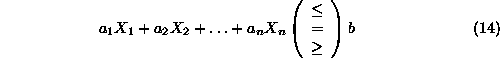and a point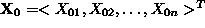satisfying Equation 14 as equality, we can perceive the solution space of

• Equation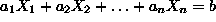as the set of points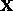for which the vector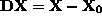is at right angles with vector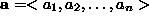,
• Inequality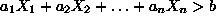as the set of pointsfor which the vectorforms an acute angle with vector, and
• Inequality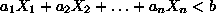as the set of pointsfor which the vectorforms an obtuse angle with vector.

From the above description, it follows that the solution space of the equation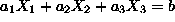, i.e., the 3-dim case, is a plane perpendicular to vector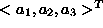, which is characterized as the plane normal. Since this normality concept carries over to the more general n-dim case, we characterize the solution space of an n-var linear equation as a hyperplane. Furthermore, similar to the 2-var LP case, a hyperplane defined by the equation, divides the n-dim space into two half-spaces: one of them is the solution space of the inequality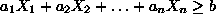, and the other one is the solution space of the inequality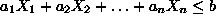.

Hence, the solution space (feasible region) of an n-var LP is geometrically defined by the intersection of a number of half-spaces and/or hyperplanes equal to the LP constraints, including the sign restrictions. Such a set is characterized as a polytope. In particular, a bounded polytope is called a polyhedron.

Two other concepts of interest in the following discussion are those of the straight line and the line segment. Given two points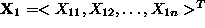and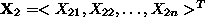, the straight line passing through them is algebraicaly defined by the set of points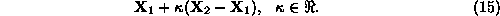Figure 7 provides a visualization of this definition.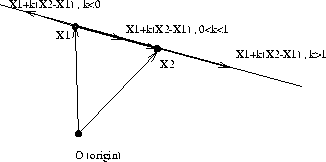Figure 7: The equation of a straight line

As it is seen in the figure above, the line segment between points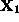and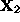is analytically defined by: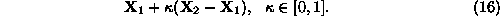Notice that Equation 16 can be rewritten as: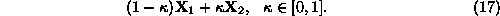or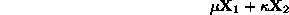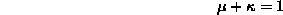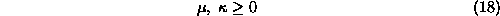We say that Equations 17 and 18 define a convex combination of pointsand.Next: Polytope Convexity and Extreme Up: Generalization to the n-var Previous: Generalization to the n-var

UAL Data
Fri Jun 20 15:03:05 CDT 1997